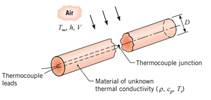### Create an Account

Home / Questions / The density and specific heat of a particular material are known ρ l200 kgm3 cp

# The density and specific heat of a particular material are known ρ l200 kgm3 cp

The density and specific heat of a particular material are known ( ρ = l200 kg/m3, cp = 1250 J/kg ∙ K), but its thermal conductivity is unknown. To determine the thermal conductivity, a long cylindrical specimen of diameter D = 40 mm is machined, and a thermocouple is inserted through a small hole drilled along the centerline.The thermal conductivity is determined by performing an experiment in which the specimen is heated to a uniform temperature of Ti = 100°C and then cooled by passing air at T = 25°C in cross flow over the cylinder. For the prescribed air velocity, the convection coefficient is h = 55 W/m2 ∙ K.

(a) If a centerline temperature of T(0, t) 40°C is recorded after t = 1136 s of cooling, verify that the material has a thermal conductivity of k 0.30 W/m ∙ K.

(b) For air in cross flow over the cylinder, the prescribed value of h = 55 W/m2 ∙ K corresponds to a velocity of V 6.8 m/s. If h CV0.618, where the constant C has units of W∙s 0.618/m2.618 ∙ K, how does the centerline temperature at t = 1136 s vary with velocity for 3 ≤ V ≤ 20 m/s? Determine the centerline temperature histories for 0 ≤ t ≤ 1500 s and velocities of 3, 10, and 20 m/s.

Jun 27 2020 View more View LessSubscribe To Get Solution